Bochner integral

(diff) ← Older revision | Latest revision (diff) | Newer revision → (diff)

An integral of a function with values in a Banach space with respect to a scalar measure. It belongs to the so-called strong integrals (cf. Strong integral).

Letbe the vector space of functions,, with values in a Banach space, given on a spacewith a countably-additive scalar measureon a-algebra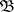of subsets of. A function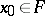is called simple if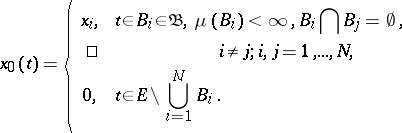A functionis called strongly measurable if there exists a sequence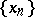of simple functions with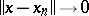almost-everywhere with respect to the measureon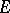. In such a case the scalar function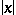is-measurable. For the simple functionA functionis said to be Bochner integrable if it is strongly measurable and if for some approximating sequenceof simple functionsThe Bochner integral of such a function over a setiswhere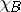is the characteristic function of, and the limit is understood in the sense of strong convergence in. This limit exists, and is independent of the choice of the approximation sequence of simple functions.

Criterion for Bochner integrability: For a strongly-measurable function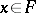to be Bochner integrable it is necessary and sufficient for the norm of this function to be integrable, i.e.The set of Bochner-integrable functions forms a vector subspace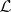of, and the Bochner integral is a linear operator on this subspace.

Properties of Bochner integrals:

1)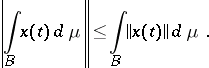2) A Bochner integral is a countably-additive-absolutely continuous set-function on the-algebra, i.e.if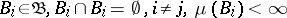, and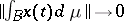if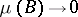, uniformly for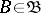.

3) If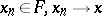almost-everywhere with respect to the measureon, ifalmost-everywhere with respect toon, and if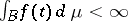, thenand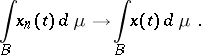4) The spaceis complete with respect to the norm (cf. Convergence in norm)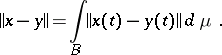5) Ifis a closed linear operator from a Banach spaceinto a Banach spaceand ifthenIfis bounded, the conditionis automatically fulfilled, .

The Bochner integral was introduced by S. Bochner . Equivalent definitions were given by T. Hildebrandt  and N. Dunford (the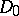-integral).

How to Cite This Entry:
Bochner integral. Encyclopedia of Mathematics. URL: http://encyclopediaofmath.org/index.php?title=Bochner_integral&oldid=11334
This article was adapted from an original article by V.I. Sobolev (originator), which appeared in Encyclopedia of Mathematics - ISBN 1402006098. See original article